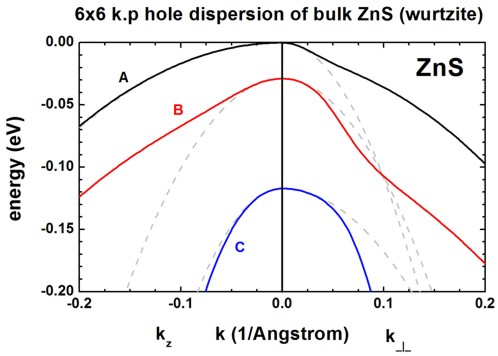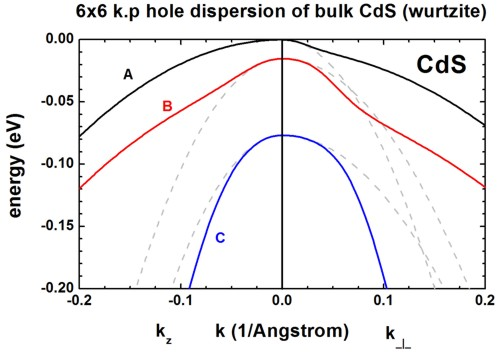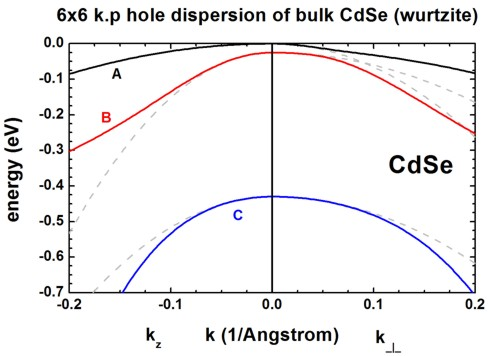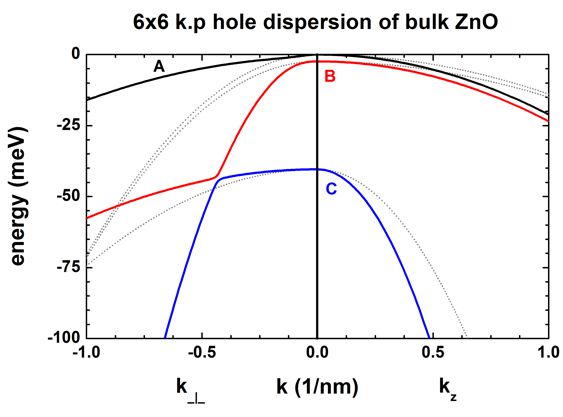nextnano.com  Tool: nextnano++  Tool: nextnano³  Download | Search | Copyright | Publications  * password protected nextnano³ software1D bulk k.p dispersion in II-VI

# nextnano3 - Tutorial

## k.p dispersion in bulk unstrained ZnS, CdS, CdSe and ZnO (wurtzite)

Author: Stefan Birner

If you want to obtain the input files that are used within this tutorial, please check if you can find them in the installation directory.
If you cannot find them, please submit a Support Ticket.
```-> bulk_6x6kp_dispersion_ZnS_nn3.in -> bulk_6x6kp_dispersion_CdS_nn3.in -> bulk_6x6kp_dispersion_CdSe_nn3.in -> bulk_6x6kp_dispersion_ZnO_nn3.in  / *_nnp.in - ```input file for nextnano3 / nextnano++ software```  ```

## k.p dispersion in bulk unstrained ZnS, CdS, CdSe and ZnO (wurtzite)

This tutorial is based on

Valence band parameters of wurtzite materials
J.-B. Jeon, Yu.M. Sirenko, K.W. Kim, M.A. Littlejohn, M.A. Stroscio
Solid State Communications 99, 423 (1996)

• We want to calculate the dispersion E(k) from |k|=0 [1/nm] to |k|=2.0 [1/nm] along the following directions in k space:
-  to , i.e. parallel to the c axis (Note: The c axis is parallel to the z axis.)
-  to , i.e. perpendicular to the c axis (Note: The (x,y) plane is perpendicular to the c axis.)
We compare 6-band k.p theory results vs. single-band (effective-mass) results.
• We calculate E(k) for bulk ZnS, CdS and CdSe (unstrained).

### Bulk dispersion along  and 

• ``` \$output-kp-data  destination-directory  = kp/  bulk-kp-dispersion     = yes  grid-position          = 5d0              ! ```in units of` [nm]```` ``````  !-----------------------------------------------------------------------------------  ! Dispersion along  direction, i.e. parallel      to c= axis in wurtzite  ! Dispersion along  direction, i.e. perpendicular to c= axis in wurtzite  ! maximum |k| vector = 2.0 [1/nm]  !-----------------------------------------------------------------------------------  k-direction-from-k-point = 0d0          0d0          2.0d0 ! ``` k-direction and range for dispersion plot``` [1/nm]  k-direction-to-k-point   = 1.41421356d0 1.41421356d0 0d0   ! ```k-direction and range for dispersion plot``` [1/nm]  ! ```The dispersion is calculated from the k point '`k-direction-from-k-point`' to Gamma, and then from the Gamma point to '`k-direction-to-k-point`'.```  number-of-k-points       = 100             ! ```number of k points to be calculated (resolution)
` \$end_output-kp-data`
• We calculate the pure bulk dispersion at` grid-position=5d0`, i.e. for the material located at the grid point at 5 nm. In our case this is ZnS but it could be any strained alloy.
In the latter case, the k.p Bir-Pikus strain Hamiltonian will be diagonalized.
The grid point at` grid-position `must be located inside a quantum cluster.
`shift-holes-to-zero = yes `forces the top of the valence band to be located at 0 eV.
How often the bulk k.p Hamiltonian should be solved can be specified via` number-of-k-points`. To increase the resolution, just increase this number.
• The maximum value of |k| is 2.0 [1/nm].
Note that for values of |k| larger than 2.0 [1/nm], k.p theory might not be a good approximation any more.
This depends on the material system, of course.
• Start the calculation.
The results can be found in:```  kp_bulk/bulk_6x6kp_dispersion_as_in_inputfile_kxkykz_000_kxkykz.dat ``` (6-band k.p)
`  kp_bulk/bulk_sg_dispersion.dat                                      `(single-band approximation)

`bulk_6x6kp_dispersion_as_in_inputfile_kxkykz_000_kxkykz.dat`:
The first column contains the |k| vector in units of [1/nm], the next six columns the six eigenvalues of the 6-band k.p Hamiltonian for this k=(kx,ky,kz) point.
The resulting energy dispersion is usually discussed in terms of a nonparabolic and anisotropric energy dispersion of heavy, light and split-off holes, including valence band mixing.

`bulk_sg_dispersion.dat`:
The first column contains the |k| vector in units of [1/nm], the next three columns the energy for heavy (A), light (B) and crystal-field split-off (C) hole  for this k=(kx,ky,kz) point.
The single-band effective mass dispersion is parabolic and depends on a single parameter: The effective mass m*.
Note that in wurtzite materials, the mass tensor is usually anisotropic with a mass mzz parallel to the c axis, and two masses perpendicular to it mxx=myy.

### Results

• Here we visualize the results.
The final figures will look like this (left: dispersion along , right: dispersion along ):• These three figures are in excellent agreement to Figure 1 of the paper by [Jeon].
• The dispersion along the hexagonal c axis is substantially different than the dispersion in the plane perpendicular to the c axis.
The effective mass approximation is indicated by the dashed, grey lines.
For the heavy holes (A), the effective mass approximation is very good for the dispersion along the c axis, even at large k vectors.

• For comparison, the single-band (effective-mass) dispersion is also shown. For ZnS, it corresponds to the following effective hole masses:
```  valence-band-masses = 0.35d0  0.35d0  2.23d0 ! [m0] ```heavy hole A  (`2.23 `along c axis)```                       0.485d0 0.485d0 0.53d0 ! [m0] ```light    hole B  (`0.53 `along c axis)```                       0.75d0  0.75d0  0.32d0 ! [m0] ```crystal hole C  (`0.32 `along c axis)``` ```The effective mass approximation is a simple parabolic dispersion which is anisotropic if the mass tensor is anisotropic (i.e. it also depends on the k vector direction).

One can see that for |k| < 0.5 [1/nm] the single-band approximation is in excellent agreement with 6-band k.p but differs at larger |k| values substantially.

• Plotting E(k) in three dimensions
Alternatively one can print out the 3D data field of the bulk E(k) = E(kx,ky,kz) dispersion.

``` \$output-kp-data  ...  bulk-kp-dispersion-3D  = yes !----------------------------------------! maximum |k| vector = 2.0 [1/nm]!----------------------------------------  k-direction-to-k-point = 0d0  0d0 2.0d0   ! ```k-direction and range for dispersion plot``` [1/nm]  number-of-k-points     = 40               ! ```number of k points to calculated (resolution)

The meaning of``` number-of-k-points = 41``` is the following:
40 k points from '```- maximum |k| vector' ```to zero (plus the Gamma point) and
40 k points from zero to  '`+ maximum |k| vector'` (plus the Gamma point) along all three directions,
i.e. the whole 3D volume then contains 81 * 81 * 81 = 531441 k points.

## k.p dispersion in bulk unstrained ZnO

The following figure shows the bulk 6-band k.p energy dispersion for ZnO.
The gray lines are the dispersions assuming a parabolic effective mass.The following files are plotted:
```- kp_bulk/bulk_6x6kp_dispersion_as_in_inputfile_kxkykz_000_kxkykz.dat - kp_bulk/bulk_sg_dispersion.dat ```The files``` - bulk_6x6kp_dispersion_axis_-100_000_100.dat ```and
`- bulk_6x6kp_dispersion_diagonal_-110_000_1-10.dat`
contain the same data because for a wurtzite crystal, due to symmetry, the dispersion in the plane perpendicular to the kz direction (corresponding to ) is isotropic.

 If you need support, or input files that are not included in the installation folder, or if you have suggestions on how we can improve our website or tutorials, please submit a Support Ticket.